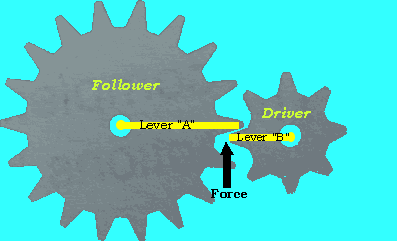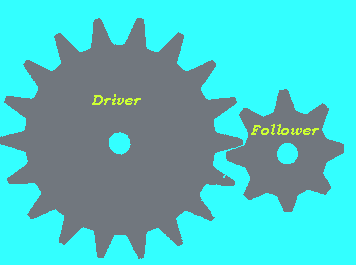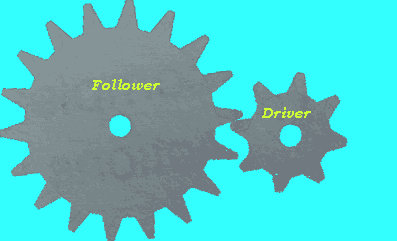Gear ratio and mechanical advantage When two gears are meshed, as in the diagram on the right, they act in a similar fashion to levers. Each gear tooth can be regarded as the end of a lever with the fulcrum placed at the centre of the gear. The longer lever "A" is, the greater the force that is applied to the shaft of the follower. We can make lever "A" longer by using gears with a geater number of teeth. We can also make lever "B" smaller, therefore increasing the force exerted at the end of the lever. Gears do not only increase speed and change the direction of motion but can multiply turning forces.The gear train on the left has a gear ratio of 8/18. If a force of 180N was applied to the driver the follower would exert a force of (8/18) X 180 = 80N. This gear train is set up to maximise speed not force. The gear train on the right has a gear ratio of 18/8. If a force of 180N was applied to the driver the follower would exert a force of (18/8) X 180 = 405N. This gear train is set up to maximise force not speed. When ever the driver is connected to a larger gear, force is multiplied. The follower rotates slowly compared to the driver but with a greater force.Home